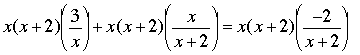SEARCH HOMEMath Central Quandaries & QueriesQuestion from Bailey, a student: 3/x + x/x+2 ______ -2/x+2Hi Bailey,

My first goal would be to eliminate the equations. The denominators are x and (x + 2) s you can eliminate the denominators by multiplying x(x+2). The equation then becomesSimplify and solve for x.

Make sure that you verify your answer,
PennyMath Central is supported by the University of Regina and The Pacific Institute for the Mathematical Sciences.